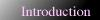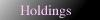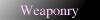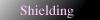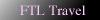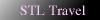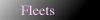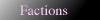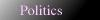The basic concepts of STL travel are covered by Newtonian physics. A constant acceleration gives x(t)=½at2+v0t+x0, where x is position, v velocity, a acceleration, and t time. This holds very well for low speeds and objects that don't move near light speed, don't shoot much propellant out the back, and don't have significant drag forces to deal with. In general, there are two cases we like to consider - least-time intercept, and zero-zero intercept. A least-time intercept means full acceleration in one direction or another, getting to the target in as little time as possible. There's one small problem with using least-time intercepts - you'll go right by the target, or through it. A zero-zero intercept calculates for starting and ending at v=0, and when reversed to calculate acceleration from time and displacement, gives twice the acceleration of a least-time intercept. If there's significant relative velocity between the two objects initially, neither model is appropriate, and one must at least solve the quadratic with the appropriate v0 term. If acceleration is variable, or the vessel experiences some drag force, a more powerful differential approach is required. When the factors approach lightspeed, Newton's model of physics no longer holds. Instead, we must work with Einstein's equations, p=γmv and E=γmc2, where γ=(1-v2/c2)-½. As γ~1 when v=0, the resting energy (mass energy) is E=mc2, while the remainder - T=(γ-1)mc2 - is kinetic. For massless particles, E=pc. In either case, momentum and total energy are conserved. There are, then, obstacles in space drives. First, any drive is limited with respect to its energy vs momentum curve. To produce momentum, you need energy, and mass. Throwing mass out the back only gives a high quantity of momentum at low speeds, which requires lots of propellant. Throwing it out fast requires lots of energy - up to 300 megajoules per 1 kgm/s of momentum on the high end case. A pure fusion rocket can theoretically develop nearly 40 million kgm/s per kg of burned fuel of thrust. This is not a significant limitation for low speeds and craft carrying adequate fuel supplies; burning 1% of your mass in hydrogen fuel and ejecting it as iron at 100% energy efficiency will get you a Δv of ~40,000 m/s; burned over a hundred seconds, that's still ~400g.